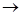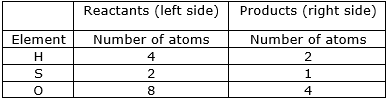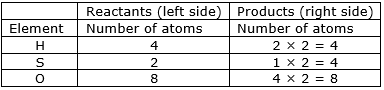### Question 17. Balance the following equation stepwise. H2S2O7(l) + H2O(l) → H2SO4(l)

Question 17.

Balance the following equation stepwise.

H2S2O7(l) + H2O(l) → H2SO4(l)

Balanced equation: H2S2O7 + H2O → 2H2SO4

Explanation:

⇒ Step 1: Write the given unbalance equation

H2S2O7 + H2O(l)H2SO4(l)

⇒ Step 2: Compare the number of atoms of reactants with the number of atoms of products.⇒ Step 3: Now, if we multiply 2 in the products, we will get the equal number of atoms as reactants.⇒ Write down the final balanced equation:

H2S2O7 + H2O → 2H2SO4